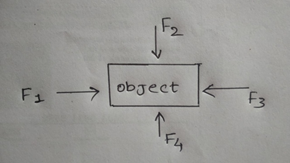Question

# Soni says that the acceleration in an object could be zero even when several forces are acting on it. Do you agree with her? Why?

Medium
SolutionVerified by Toppr

## When equal and opposite forces act on any object, then they cancel out each other so that the total external force experienced by the object is zero.So net force, Where, F=Net force, m=Mass, a=Acceleration.Hence, If net force (F) =0, then acceleration (a) will also be equals to zero (because mass ‘m’ cannot be zero). Therefore, acceleration in an object could be zero even when several forces are acting on it. Hence, Agree with Soni.

Video Explanation00